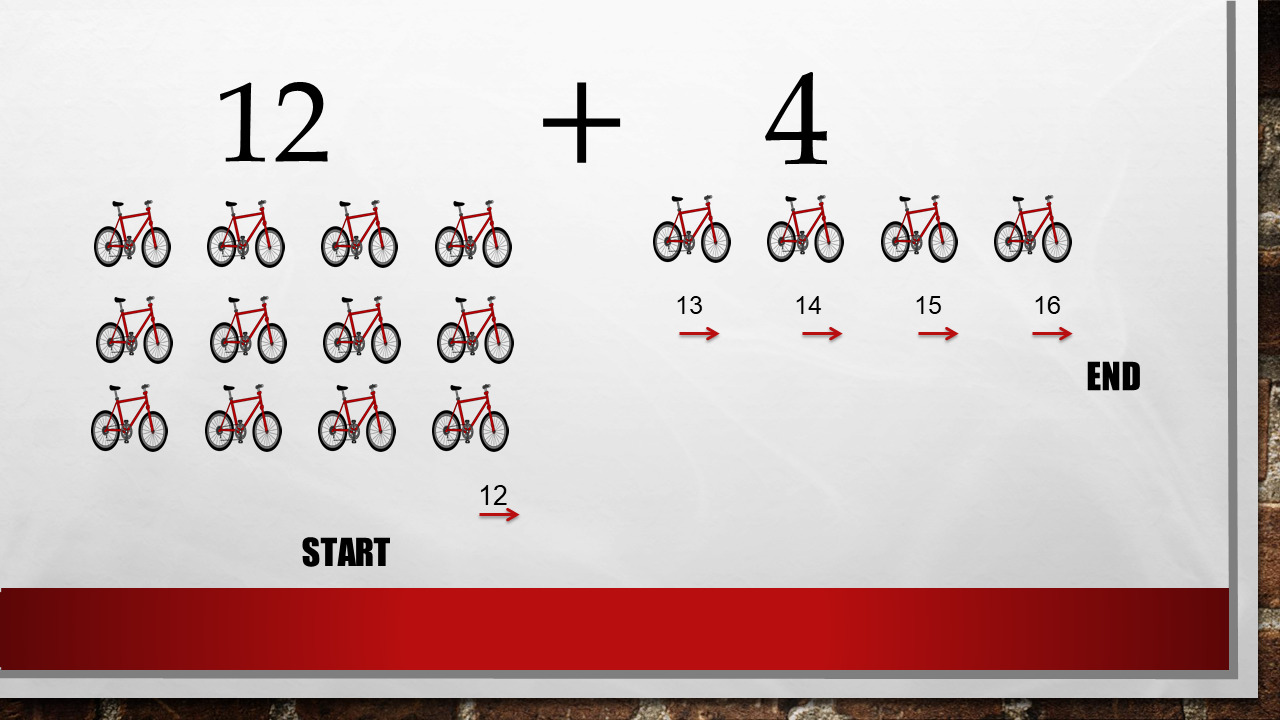1
visibility

Find the sum of the given numbers: 4 + 12

• A

15

• B

16

• C

17

• D

18

The question is exactly 12 + 4 = ?

Now, we can count from any number. Count on!

12 + 4 = ?

We count  4 numbers from 12.

From 12, 13, 14, 15, 16

So: 4 + 12 = 16